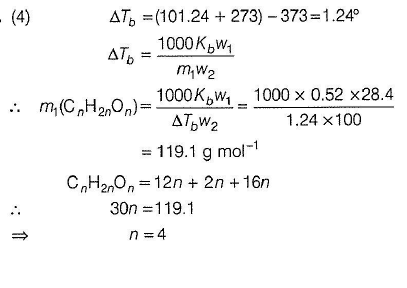Courses

# Test: Colligative Properties III (Elevation In Boiling Point)

## 20 Questions MCQ Test Chemistry Class 12 | Test: Colligative Properties III (Elevation In Boiling Point)

Description
This mock test of Test: Colligative Properties III (Elevation In Boiling Point) for Class 12 helps you for every Class 12 entrance exam. This contains 20 Multiple Choice Questions for Class 12 Test: Colligative Properties III (Elevation In Boiling Point) (mcq) to study with solutions a complete question bank. The solved questions answers in this Test: Colligative Properties III (Elevation In Boiling Point) quiz give you a good mix of easy questions and tough questions. Class 12 students definitely take this Test: Colligative Properties III (Elevation In Boiling Point) exercise for a better result in the exam. You can find other Test: Colligative Properties III (Elevation In Boiling Point) extra questions, long questions & short questions for Class 12 on EduRev as well by searching above.
QUESTION: 1

### Only One Option Correct Type This section contains 13 multiple choice questions. Each question has four choices (a), (b), (c) and (d), out of which ONLY ONE is correct Elevation in boiling point of an aqueous solution of a non-electrolyte solute is 1.04°. What is the depression in freezing point of this solution? Kf (H20) = 0.52° mol-1 kg Kf (H20) = 1.86° mol-1 kg

Solution: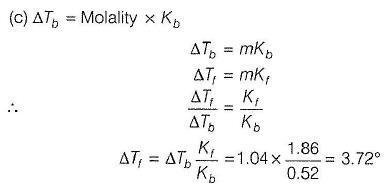QUESTION: 2

### 12.8 g of naphthalene (C10H8) is dissolved in 92 g toluene (C7H8). Boiling point of this solution is (boiling point of toluene = 110°Cand mole fraction boiling point elevation constant of toluene is 36.1 K)

Solution:

When (Kz) mole fraction boiling point elevation constant is given, then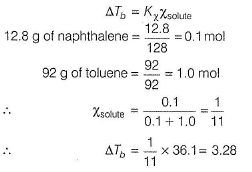Thus,boiling point of solution =110+ 3.28 =113.28°C

QUESTION: 3

### Elevation in boiling point of a molar (1 M) glucose solution (d = 1.2 g mL-1) is

Solution:

(a) Molarity =1 M =1 mol L-1

1000 mL of solution has glucose = 180 g

(1000 x 1.2) g of solution has glucose = 180g

∴ Solute (w1) =180 g

Solution =1200 g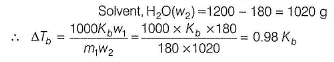QUESTION: 4

For a dilute solution containing 2.5 g of a non-volatile non-electrolyte solute in 100 g water, the elevation in boiling point at 1 atm pressure is 2°C. Assuming concentration of solute is much lower than the concentration of solvent, the vapour pressure (mm of Hg) of the solution is (Take, Kb = 0.76 K mol-1 kg).

[IITJEE2012]

Solution: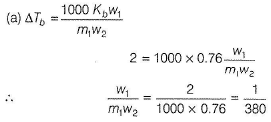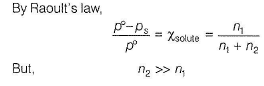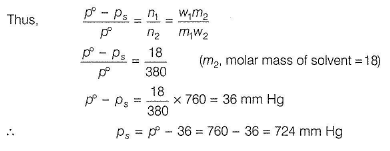QUESTION: 5

Relative lowering of vapour pressure of a non-volatile solute X in water is 0.05. If   Kb(H20) = 0.52° mol-1  kg-1.

(ΔTb) = elevation in boiling point assuming a dilute solution

(ATb)' = elevation in boiling point assuming a solution of moderate concentration then, {ΔTb) - {ΔTb)' =

Solution:

By Raoult’s law,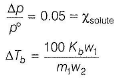If solution is dilute,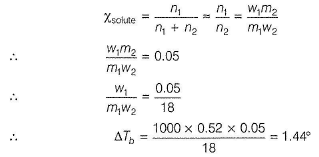If solution is of moderate concentration,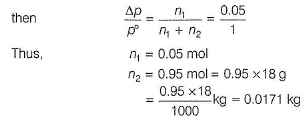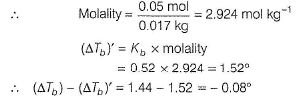QUESTION: 6

6% aqueous solution of urea (by mass of solvent) has elevation in boiling point xt. 18% aqueous solution of glucose (by mass of solvent) has elevation in boiling point x2. If these two solutions are mixed then, elevation in boiling point is x3. Then,

Solution: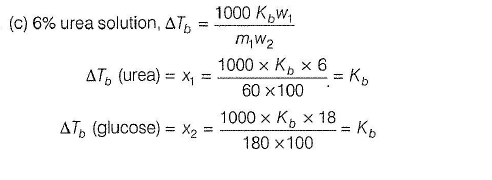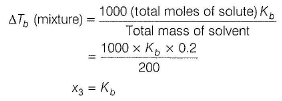Thus,  x1 =x2 = x3

QUESTION: 7

AB is a sparingly soluble salt in aqueous solution with Ksp =1x10-8. If Kb(H20 ) = 0.52° mol-1 kg then elevation in boiling point of saturated solution is

Solution:

(a) Salt is sparingly soluble hence, can be considered as non-electrolyte.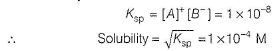Since, solubility is very low, molarity can be taken as molality.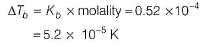QUESTION: 8

Elevation in boiling point of a solution of non-electrolyte in non-polar solvent (toluene) is 0.3°. Mole fraction elevation constant of non-polar solvent is 36.1 K. Thus, molality (mol kg-1) of the solution is

Solution:

(b) In terms of mole fraction elevation constant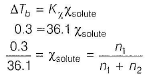Thus, solute = 0.3 mole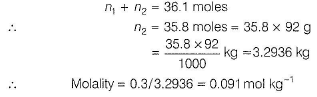QUESTION: 9

Which of the given aqueous solution has maximum boiling point?

(Kb of H20 = 0 .5 2 ° mol-1 kg), Tb (H20 ) = 373 K)

Solution: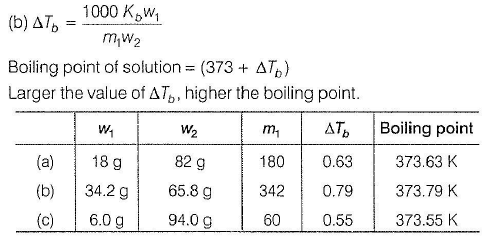QUESTION: 10

X and Y both are soluble in given solvent. Molar mass of the polymer Y is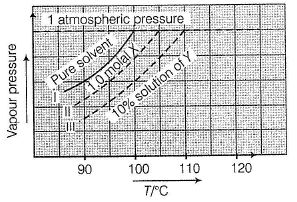Solution: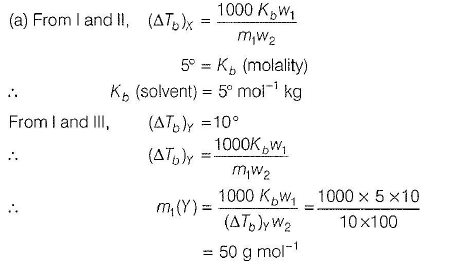QUESTION: 11

Given, H20 ( / )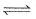H20 ( g ) a t 373 K, ΔH° = 9.72 kcal mol-1 and the concentration of the sucrose in water is 0.1 M. Find the elevated boiling point of water.

Solution:

(b) H20 (/) changes to steam FlaO (g) at 373 K. Thus, this represents latent heat of vaporisation. Kb (molal elevation constant) is related to ΔH° and boiling point by equation,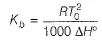Here, ΔH° is in energy unit per gram of solvent.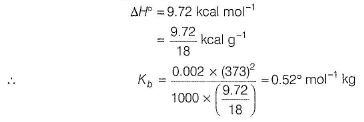ΔTb (sucrose solution) = molality x Kb - 0.1 x 0.52 = 0.052°

∴Boiling point of solution = 373 + 0.052° = 373.052 K

QUESTION: 12

Glucose solution is 20% by weight of solution. Its boiling point is ........
Given 1 molal urea solution boils at 100.52° C and water boils at 1 atm pressure,

Solution:

(d)

Water boils at 1 atmospheric pressure. Thus, boiling point of H20 =100°C

1 molal urea solution boils at 100.52°C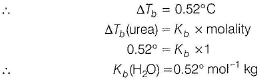Glucose solution is 20% by weight of solution.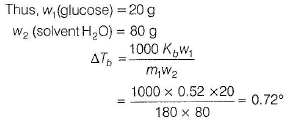∴Boiling point of glucose solution = 100.720C

QUESTION: 13

Latent heat of fusion and latent heat of vaporisation of a solvent are in the ratio of 1: 6 and freezing point and boiling point (in Kelvin scale) are in the ratio of 2:3. Thus, ratio of elevation in boiling point to depression in freezing point of 1 molal solution is

Solution:

(d) Kb (molal elevation constant) is related to latent heat of vaporisation (ΔHV)and boiling point (Tb)of solvent by relation.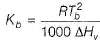Similarly, Kf (molal depression constant) is related to latent heat of fusion ((ΔHf) and boiling point 7, of solvent by relation.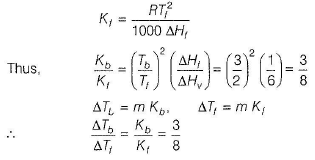*Multiple options can be correct
QUESTION: 14

One or More than One Options Correct Type
This section contains 2 multiple type questions. Each question has 4 choices (a), (b), (c) and (d), out of which ONE or MORE THAN ONE are correct.

Q. Out of the following, colligative properties are

Solution:

Any property which is dependent on the number of the particles of solute in a solution, is called colligative property. (a) Osmotic pressure,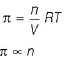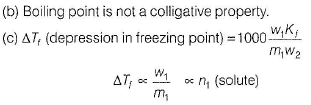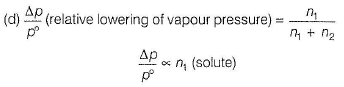If there is change in number of moles of solute, the following properties also change: (i) If solute is non-electrolyte, equal moles will have same property.

(ii) There is ionisation, n changes hence, properties change.

(iii) There is polymerisation, n changes hence, properties change.

*Multiple options can be correct
QUESTION: 15

10% aqueous solution of a non-electrolyte solute of molar mass (m) has elevation in boiling point x°, then

Solution:

(a,b,c,d)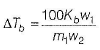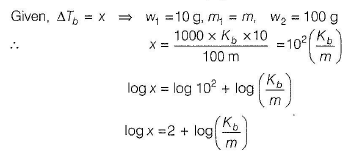This represents linear equation.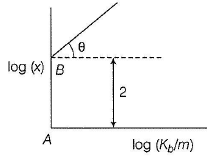With slope =1 = tan θ (θ = 45°) Intercept, AB =2

As log (x) increases, log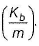also increases. Hence, X is K m ) dependent on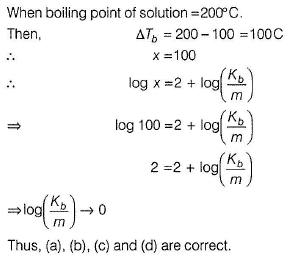QUESTION: 16

Comprehension Type
This section contains a passage describing theory, experiments, data, etc. Two questions related to the paragraph have been given. Each question has only one correct answer out of the given 4 options (a), (b), (c) and (d)

Passage

Heavy water boils at 101.42°Cand its molal elevation constant ( Kb ) is 10% higher than that of pure water. Elevation in 0.1 molal aqueous urea solution is 0.052°.

Q. What is the molal elevation constant (in K mol-1 kg) of heavy water?

Solution:

For aqueous solution of urea,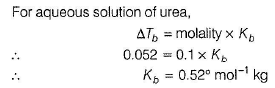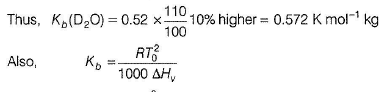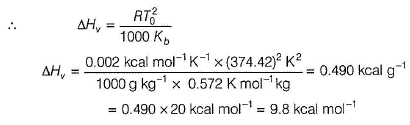QUESTION: 17

What is the latent heat of vaporisation of heavy water?

Solution:

For aqueous solution of urea,QUESTION: 18

Matching List Type
Choices for the correct combination of elements from Column I and Column II are given as options (a), (b), (c) and (d), out of which one is correct

Q. One molal aqueous sucrose solution boils at 100.52° C and water boils at 100° C. 5% aqueous solution (by mass of solvent) of non-electrolyte A B 2 boils at 100.26° C, 9% aqueous solution (by mass of solvent) of non-electrolyte A 2B boils at 100.52°C. Match the molar masses given in Column II with the species in Column l and select the answer from the codes given below.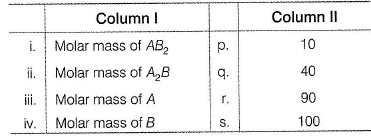Codes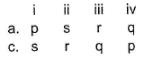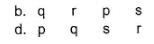Solution: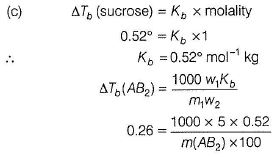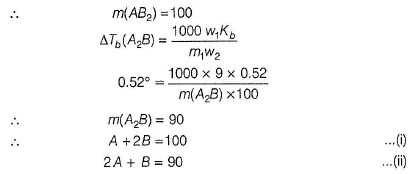On solving Eqs. (i) and (ii), we get A = 40
6=10
Hence, (i) →(s), (ii) → (r), (iii)→ (q), (iv) →(p)

*Answer can only contain numeric values
QUESTION: 19

One Integer Value Correct Type
This section contains 2 questions, when worked out will result in an integer value from 0 to 9 (both inclusive)

Q. A solution containing 3.975 g of sulphur in 100 g ofCS2 boils at 319.67 K. The boiling point of pure CS2 is 319.30 K.
Kb( CS2 ) = 2.406° mol-1 kg
If molecular state of sulphur is (S)x then what is the value of x?

Solution: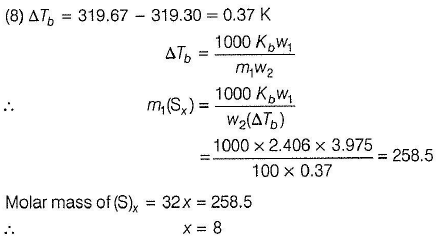*Answer can only contain numeric values
QUESTION: 20

An aqueous solution containing 28.4 g of non-volatile compound having the stoichiometric composition CnH2nOn in 100 g water boils at 101.24°Cat 1 atm pressure. Kf (H20 ) = 0.52° mol-1 kg and boiling point of H20 = 373 K. What is the value of n?

Solution: